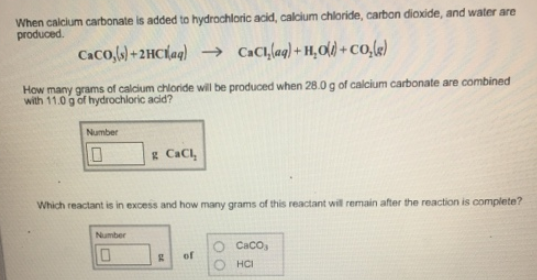# Problem: When calcium carbonate is added to hydrochloric acid, calcium chloride, carbon dioxide, and water produced. CaCO3(s) + 2HCl(aq) → CaCl2(aq) + H2O(l) + CO2(g)How many grams of calcium chloride wll be produced when 28.0 g of calcium carbonate are combined with 11.0 g of hydrochloric acid? Which reactant is in excess and how many grams of this reactant will remain after the reaction is complete?

###### FREE Expert Solution
100% (325 ratings)###### Problem Details

When calcium carbonate is added to hydrochloric acid, calcium chloride, carbon dioxide, and water produced.

CaCO3(s) + 2HCl(aq) → CaCl2(aq) + H2O(l) + CO2(g)

How many grams of calcium chloride wll be produced when 28.0 g of calcium carbonate are combined with 11.0 g of hydrochloric acid?

Which reactant is in excess and how many grams of this reactant will remain after the reaction is complete?